WORKING WITH METERS

All You Will Need to Know About Metric

------------------------------------------------------------
10 Metric is based on the Decimal system

The metric system is simple to learn. For use in your everyday life you will need to know only ten units. You will also need to get used to a few new temperatures. Of course, there are other units which most persons will not need to learn. There are even some metric units with which you are already familiar; those for time and electricity are the same as you use now.

------------------------------------------------------------
BASIC UNITS
 Meter: a little longer than a yard (about 1.1 yards)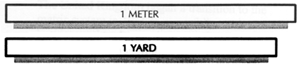Liter: a little larger than a quart (about 1.06 quarts) Gram: a little more than the weight of the paper clip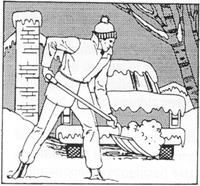25 Degrees Fahrenheit

COMMON PREFIXES
(to be used with basic units)
milli: one-thousandth (0.001)
centi:one-hundredth (0.01)
kilo: one-thousand times (1000)

For example:
1000 millimeters = 1 meter
100 centimeters = 1 meter
1000 meters = 1 kilometer

##### 1 LITER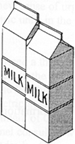##### 1 QUART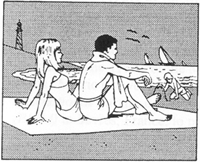25 Degrees Celsius
OTHER COMMONLY USED UNITS
millimeter: 0.001 meter - diameter of a paper clip wire
centimeter: 0.01 meter - a little more than the width of a paper clip (about 0.4 inch)
kilometer: 1000 meters - somewhat further than 1/2 mile (about 0.6 miles)
kilogram: 1000 grams - a little more than 2 pounds (about 2.2 pounds)
milliliter: 0.001 liter - five of them make a teaspoon

OTHER USEFUL UNITS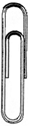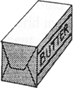1 pound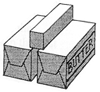1 Kilogram

A meter is a measure of length or distance.
A
meter is a little bit longer than a yard.

Name the sport items below that are about one meter long.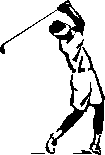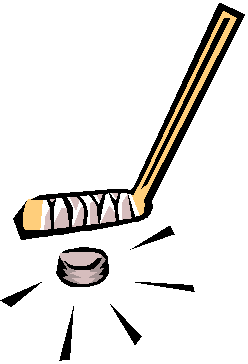Here are some other metric measures for length that are used often:

 MILLIMETER CENTIMETER KILOMETER

Here are some items that are one millimeter wide.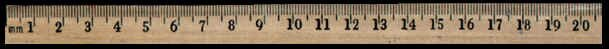(Ruler is close to actual size on an 800 X 600 screen.)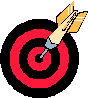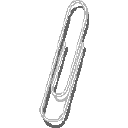The width of the The width of the point of the The thickness of the  point of a pen The width of the wire of a

Here are some items that are one centimeter long or wide.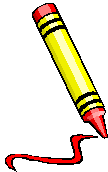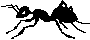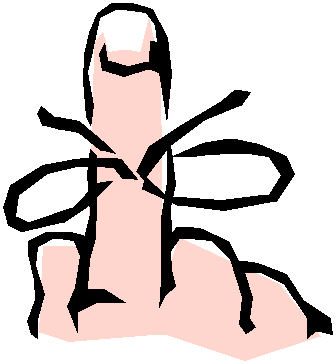The width of a The length of ant The width of a nail on your

Here are some items that are one kilometer long or wide.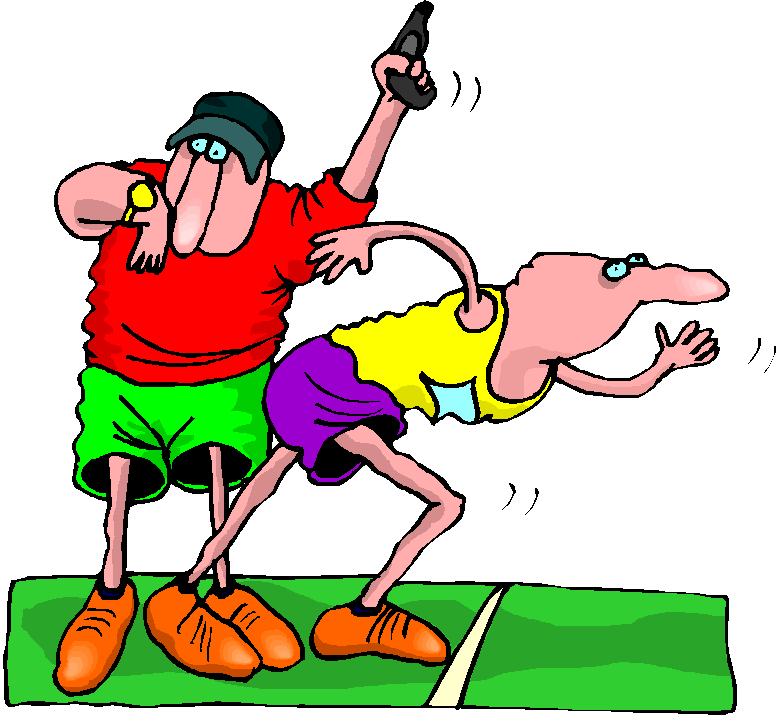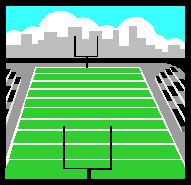2 TRIPS AROUND THE HIGH SCHOOL TRACK 11 FOOTBALL FIELDS LINED UP DISTANCE FROM EVG TO THE EDGEWATER LIBRARY

NOW  IT'S TIME TO LEARN HOW TO USE A METRIC RULER.
The ruler below is a centimeter ruler.  It is more than 4cm long.

10 millimeters  =  1 centimeter    (Ruler is not actual size.)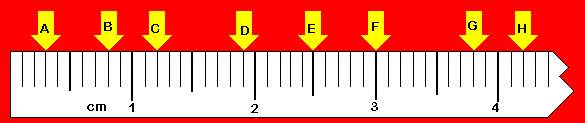To practice working with kilometers we can't use a ruler.
1000 meters = 1 kilometer

Millimeters and Centimeter Practice